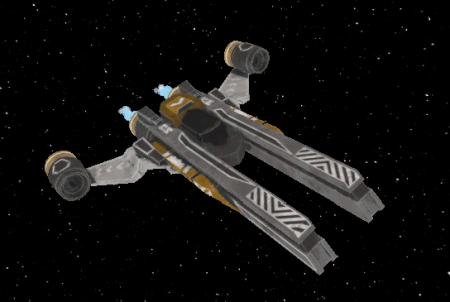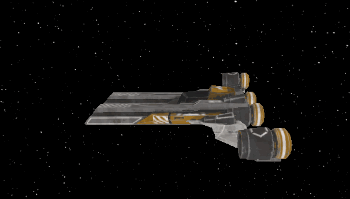## Problem

You want to make a 3D spaceship that flies in an arcade/cinematic way. You’re not looking for realistic physics, but more of a dog-fighting, “Star Wars”-style of spaceflight.

## Solution

To accomplish this, we’ll use a `KinematicBody` for the ship. The three axis inputs (pitch, roll, and yaw) will rotate the body’s `basis` around the corresponding axis. The direction of motion will always point forward.

### Assets

Spaceship models are from this asset pack:

Ultimate Spaceships Pack by Quaternius

I’ve chosen the “Executioner” ship model:Feel free to choose your favorite design.

### Setup

Select the `gltf` file of the ship you want, and click the Import tab. Change the Root Type to `KinematicBody` and click “Reimport”. Then double-click the `gltf` and you’ll have a new inherited scene with a `KinematicBody` root and a `MeshInstance` child. Add a `CollisionShape` to the body.

In Project Settings -> Input Map, set up the following inputs:

• `roll_right `/ `roll_left`
• `pitch_up` / `pitch_down`
• `yaw_right` / `yaw_left`
• `throttle_up` / `throttle_down`

You can assign keys or controller inputs. Analog stick inputs will work best.

### Movement

To start the script, let’s handle the forward movement. Pressing the throttle buttons smoothly increases/decreases the speed.

``````extends KinematicBody

export var max_speed = 50
export var acceleration = 0.6

var velocity = Vector3.ZERO
var forward_speed = 0

func get_input(delta):
if Input.is_action_pressed("throttle_up"):
forward_speed = lerp(forward_speed, max_speed, acceleration * delta)
if Input.is_action_pressed("throttle_down"):
forward_speed = lerp(forward_speed, 0, acceleration * delta)

func _physics_process(delta):
get_input(delta)
velocity = -transform.basis.z * forward_speed
move_and_collide(velocity * delta)
``````

Make a test scene with a `Camera` to try it out. You can use a stationary camera or a chase camera. Check that the ship accelerates and slows before moving on to the next step.### Rotation

Now we can handle rotation in the three axes. Add the following variables at the top of the script:

``````export var pitch_speed = 1.5
export var roll_speed = 1.9
export var yaw_speed = 1.25

var pitch_input = 0
var roll_input = 0
var yaw_input = 0
``````

The three axis speeds will affect the “handling” of the ship. Experiment to find values the work for you and your desired flight style.

Next, add these lines to `get_input()` to capture the three axis inputs:

``````pitch_input = Input.get_action_strength("pitch_up") - Input.get_action_strength("pitch_down")
roll_input = Input.get_action_strength("roll_left") - Input.get_action_strength("roll_right")
yaw_input = Input.get_action_strength("yaw_left") - Input.get_action_strength("yaw_right")
``````

Finally, we need to rotate the ship’s `Basis` according to the inputs. Note how each input affects one axis of rotation:

``````transform.basis = transform.basis.rotated(transform.basis.z, roll_input * roll_speed * delta)
transform.basis = transform.basis.rotated(transform.basis.x, pitch_input * pitch_speed * delta)
transform.basis = transform.basis.rotated(transform.basis.y, yaw_input * yaw_speed * delta)
transform.basis = transform.basis.orthonormalized()
``````### Improvements

Currently the rotations are a little to “sharp”. The ship starts and stops rotating instantly, which feels a bit too unnatural. We can solve this with `lerp()`, and by adding one more configuration variable to set how “floaty” we’d like the controls to be:

``````export var input_response = 8.0
``````

Change the three axis inputs in `get_input()` to the following:

``````pitch_input = lerp(pitch_input,
Input.get_action_strength("pitch_up") - Input.get_action_strength("pitch_down"),
input_response * delta)
roll_input = lerp(roll_input,
Input.get_action_strength("roll_left") - Input.get_action_strength("roll_right"),
input_response * delta)
yaw_input = lerp(yaw_input,
Input.get_action_strength("yaw_left") - Input.get_action_strength("yaw_right"),
input_response * delta)
``````

Now when stopping or changing direction, there’s a little bit of inertia.One problem with this control scheme is that it’s awkward. Having to use a separate stick for the yaw input makes it difficult to control, especially when also shooting and using other controls. Many games solve this by linking the roll input to also apply a small amount of yaw. To do this, change the `yaw_speed` to around 1/4 to 1/2 of the `roll_speed`.

In the `get_input()` function, change the line getting `yaw_input` to the following:

``````yaw_input = roll_input
``````

This is another fun place to experiment by changing the roll and yaw speeds. For example, what if yaw was primary and roll smaller? What if other axes were linked? If your game has different ships, you can give them different values for variety in flight styles/performance.

### Wrapping up

That’s it, now you can fly! This controller is a great start for whatever space-based game you might have in mind. Add some other ships, and a few effects, and you’re ready go:

### Full script

Here’s the complete script:

``````extends KinematicBody

export var max_speed = 50
export var acceleration = 0.6
export var pitch_speed = 1.5
export var roll_speed = 1.9
export var yaw_speed = 1.25  # Set lower for linked roll/yaw
export var input_response = 8.0

var velocity = Vector3.ZERO
var forward_speed = 0
var pitch_input = 0
var roll_input = 0
var yaw_input = 0

func get_input(delta):
if Input.is_action_pressed("throttle_up"):
forward_speed = lerp(forward_speed, max_speed, acceleration * delta)
if Input.is_action_pressed("throttle_down"):
forward_speed = lerp(forward_speed, 0, acceleration * delta)

pitch_input = lerp(pitch_input,
Input.get_action_strength("pitch_up") - Input.get_action_strength("pitch_down"),
input_response * delta)
roll_input = lerp(roll_input,
Input.get_action_strength("roll_left") - Input.get_action_strength("roll_right"),
input_response * delta)
yaw_input = lerp(yaw_input,
Input.get_action_strength("yaw_left") - Input.get_action_strength("yaw_right"),
input_response * delta)
# replace the line above with this for linked roll/yaw:
# yaw_input = roll_input

func _physics_process(delta):
get_input(delta)
transform.basis = transform.basis.rotated(transform.basis.z, roll_input * roll_speed * delta)
transform.basis = transform.basis.rotated(transform.basis.x, pitch_input * pitch_speed * delta)
transform.basis = transform.basis.rotated(transform.basis.y, yaw_input * yaw_speed * delta)
transform.basis = transform.basis.orthonormalized()
velocity = -transform.basis.z * forward_speed
move_and_collide(velocity * delta)
``````